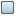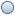AnyLogic

# Dynamic systems modeling

Dynamic Systems modeling is actually the ancestor of System Dynamics modeling approach. It is used in mechatronic, electrical, chemical, and other technical engineering disciplines as a standard part of the design process. The underlying mathematical model of a dynamic system would consist of a number of state variables and algebraic differential equations of various forms over these variables. In contrast with the System Dynamics, integrated variables here have some "physical" meaning: location, speed, acceleration, pressure, concentration, etc., they are inherently continuous, and are not aggregates of some agents.

Along with System Dynamics, Discrete Event and Agent Based modeling approaches, AnyLogic provides handy tools for Dynamic Systems approach:

• AnyLogic provides dynamic variables for defining differential and algebraic equations:
•Stock Variable - for differential equations
•Dynamic Variable - for formulas
• AnyLogic provides a set of numerical methods for solving ordinal differential equations, algebraic-differential equations, or algebraic equations. AnyLogic chooses the numerical solver automatically at runtime in accordance to the behavior of the system. When solving ordinal differential equations, it starts integration with forth-order Runge-Kutta method with fixed step. Otherwise, AnyLogic plugs in another solver – Newton method. This method changes the integration step to achieve the given accuracy.
• AnyLogic supports modeling physical systems using Finite Element method (you can find the method description here: ). In this case arrays and dimensions of range type are used extensively and you can refer next and previous array elements in equations. Demo model: Vibrating String
• Moreover, AnyLogic is the only existing tool, where continuous and discrete behaviors are two parts of the same language, are defined together and can interoperate naturally. You can make your model hybrid by adding discrete events, that will affect the continuous behavior of the model, e.g. you can add a condition event monitoring continuous variables and performing some action when some variable reaches the defined threshold, or an event, that changes a parameter of an equation and thus affects the dynamic system being modeled.
• You can expose variables to an interface of the agent and connect them with interface variables of other agents. Connected variables will have the same value at any moment of time; i.e., this mechanism provides for continuous time object interaction. This enables AnyLogic users to create blocks equivalent to the ones mostly used in block diagrams - typical graphical modeling language that control engineers use. Demo model: Population